# C# Tutorial – Create a Pong arcade game in Visual Studio

In this tutorial we will learn how to create a fully functional Pong game in visual studio using c# programming language. We will use Windows Form Application to make this game. Player has up and down key board controls and we have an opponent controlled by the CPU and we will program it in the code. We will be using Visual Studio 2010 version however this will work with any other new version of Visual Studio.

Lesson outcomes –

1. Create a fully working pong arcade game
2. Collision detection between player and CPU
3. Using picture boxes and its properties in C#
4. Using timer component to animate the ball, player and opponent
5. Create a boundary limit for the player and CPU
6. Keep score for both player and CPU
7. Increase the ball speed when either of the players score in the game

Demo:

Start Visual studio, create a new project, under C# programming language choose Windows Form Application and name project pong and click OK.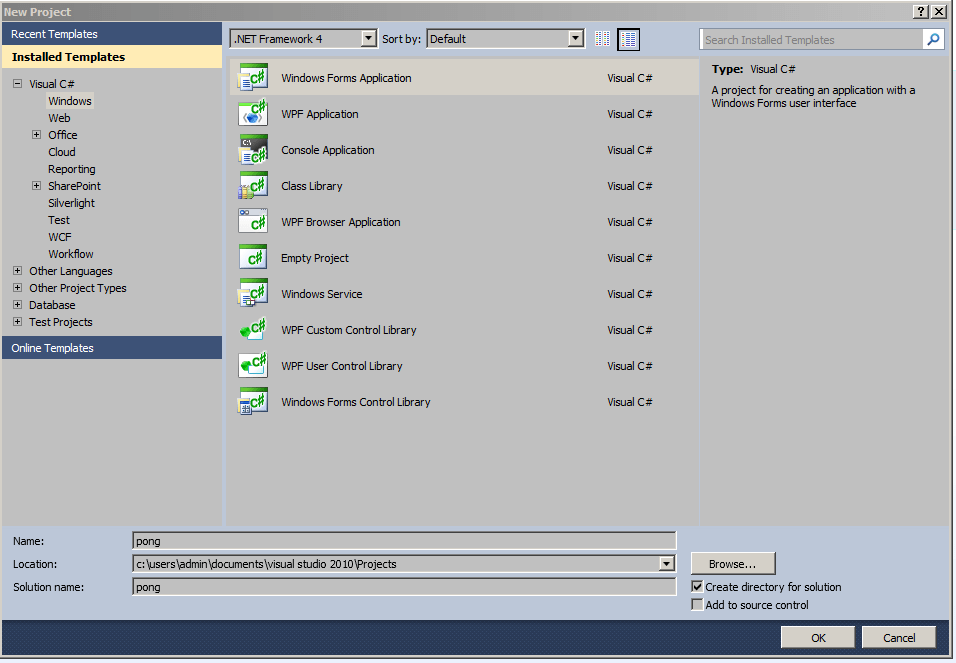Click OK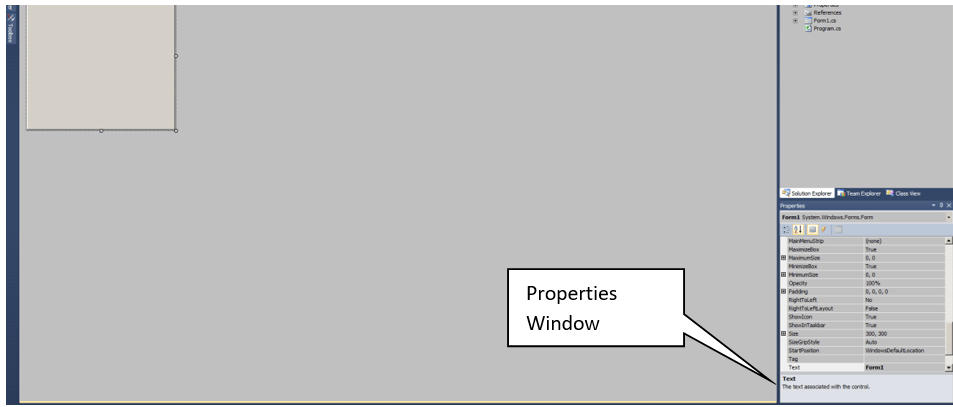In the properties windows change the following for the form

Backcolor – MidnightBlue

Size – 944, 613

Text – Pong Game MOO ICT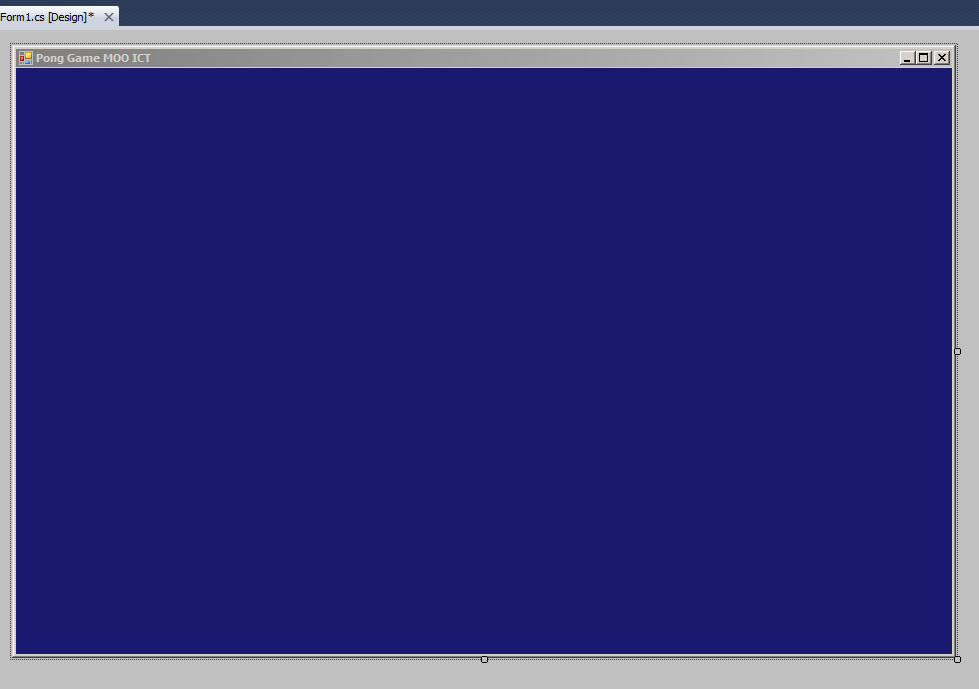This is what the form looks like now after we made the changes in the properties.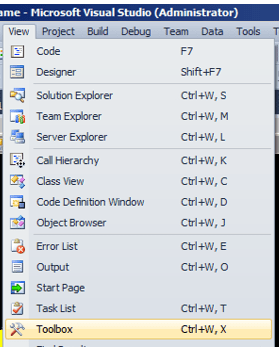We need the tool box, if the tool box isn’t present then go to View -> Click ok ToolboxFind the picture box from the tool box and place 3 of them on the form

Set their properties to the followingNow from the tool box drag and drop a timer object to the form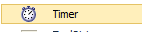Change the following to the timer properties. Change the name to gameTimer, change enabled to True and interval to 20.

Drag and drop 2 labels to the form from the toolbox. Change the following to these labels in the properties window.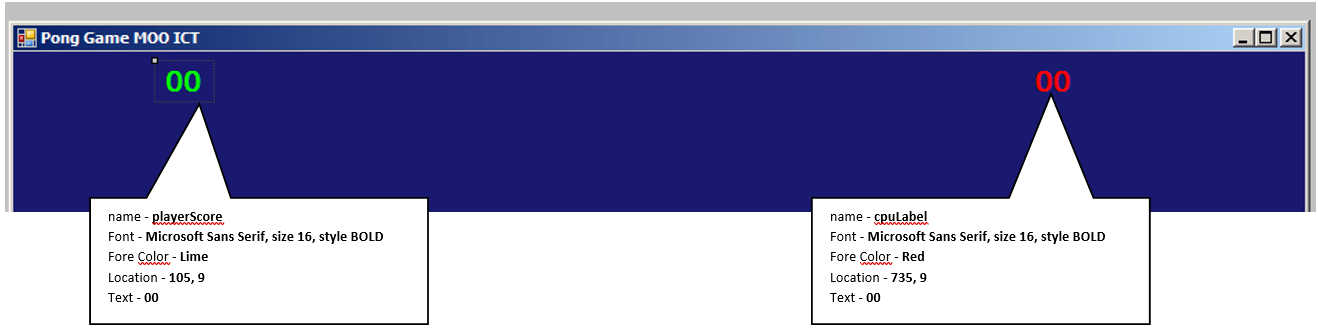With all this doneThis is the final version of the form thus far.

Click on the Form and go to the events menu in the properties window.

Click on the little lightning bolt icon in the properties window to see the events menu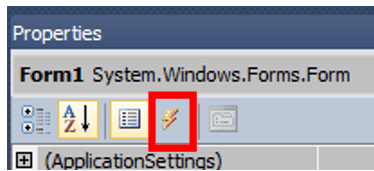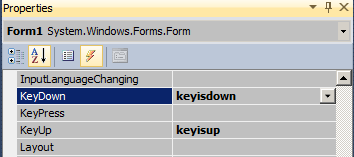Find the key down and key up option, type keyisdown for key down and keyisup for key up event. Press enter after each, this will take you to the code view. For now come back to the design view we need add one more event for the timer.

Click on the timer object and go to the events window for the timer objectType in timerTick and press enter. This will take you back to the code view. Now we can get to programming the game.

```using System;
using System.Collections.Generic;
using System.ComponentModel;
using System.Data;
using System.Drawing;
using System.Linq;
using System.Text;
using System.Windows.Forms;

namespace pong
{
public partial class Form1 : Form
{
public Form1()
{
InitializeComponent();
}

private void keyisdown(object sender, KeyEventArgs e)
{

}

private void keyisup(object sender, KeyEventArgs e)
{

}

private void timerTick(object sender, EventArgs e)
{

}
}
}```

These are events that we added to the game so far.

Now we will add a few variables to the game. All of these variables will be global variables so we need to declare them on top of the public Form1() line

```        bool goup; // boolean to be used to detect player up position
bool godown; // boolean to be used to detect player down position
int speed = 5; // integer called speed holding value of 5
int ballx = 5; // horizontal X speed value for the ball object
int bally = 5; // vertical Y speed value for the ball object
int score = 0; // score for the player
int cpuPoint = 0; // score for the CPU
```

// all the green lines are comments they can be inserted into the code for easy reading and keeping notes on the code. All of the codes will be explained in the green text.

We have several variables for this game.

Bool stands for Boolean we have two of them in the code above. GOUP and GODOWN both Booleans are set the false by default these will be used to check when to move the player up or down the screen.

INT stands for integer. We have SPEED, BALLX, BALLY, SCORE and CPUPOINT as integers. Each of the has their own value for example BALLX will determine the horizontal speed of the ball and BALLY will determine the vertical speed of the ball.

key down event

```        private void keyisdown(object sender, KeyEventArgs e)
{
if (e.KeyCode == Keys.Up)
{
// if player presses the up key
// change the go up boolean to true
goup = true;
}
if (e.KeyCode == Keys.Down)
{
// if player presses the down key
// change the go down boolean to true
godown = true;
}
}```

In this KEYISDOWN function we have two if statement. In each we are checking if that given key is down for example if the player presses and holds the UP key then the GOUP Boolean becomes true so does the for the down key.

Key up event

```        private void keyisup(object sender, KeyEventArgs e)
{
if (e.KeyCode == Keys.Up)
{
// if player leaves the up key
// change the go up boolean to false
goup = false;
}
if (e.KeyCode == Keys.Down)
{
// if player leaves the down key
// change the go down boolean to false
godown = false;
}
}```

In this KEYISUP function if the player leaves that key then we can change those Booleans to false. This event will only run when the key is pressed and released.

Timer Tick event function. In this function all the code will run during the timer ticks, we set the timer to run every 20 milliseconds so all of these code will be loaded during that time. This gives us a change to check for collision, move the ball, animate the player, animate the CPU and update the score or even end the game. All of the code below are commented so please read them through.

```        private void timerTick(object sender, EventArgs e)
{
// this is the main timer event, this event will trigger every 20 milliseconds

playerScore.Text = "" + score; // show player score on label 1
cpuLabel.Text = "" + cpuPoint; // show CPU score on label 2

ball.Top -= bally; // assign the ball TOP to ball Y integer
ball.Left -= ballx; // assign the ball LEFT to ball X integer

cpu.Top += speed; // assignment the CPU top speed integer

// if the score is less than 5
if (score < 5)
{
// then do the following

// if CPU has reached the top or gone to the bottom of the screen
if (cpu.Top < 0 || cpu.Top > 455)
{
//then
//change the speed direction so it moves back up or down
speed = -speed;
}
}
else
{
// if the score is greater than 5
// then make the game difficult by
// allowing the CPU follow the ball so it doesn't miss
cpu.Top = ball.Top + 30;
}

//check the score
// if the ball has gone pass the player through the left
if (ball.Left < 0)
{
//then
ball.Left = 434; // reset the ball to the middle of the screen
ballx = -ballx; // change the balls direction
ballx -= 2; // increase the speed
cpuPoint++; // add 1 to the CPU score
}

// if the ball has gone past the right through CPU

if (ball.Left + ball.Width > ClientSize.Width)
{
// then
ball.Left = 434;  // set the ball to centre of the screen
ballx = -ballx; // change the direction of the ball
ballx += 2; // increase the speed of the ball
score++; // add one to the players score
}

//controlling the ball
// if the ball either reachers the top of the screen or the bottom
if (ball.Top < 0 || ball.Top + ball.Height > ClientSize.Height)
{
// then
//reverse the speed of the ball so it stays within the screen
bally = -bally;
}

// if the ball hits the player or the CPU
if (ball.Bounds.IntersectsWith(player.Bounds) || ball.Bounds.IntersectsWith(cpu.Bounds))
{
// then bounce the ball in the other direction
ballx = -ballx;
}

// controlling the player

// if go up boolean is true and player is within the top boundary
if (goup == true && player.Top > 0)
{
// then
// move player towards to the top
player.Top -= 8;
}

// if go down boolean is true and player is within the bottom boundary
if (godown == true && player.Top < 455)
{
// then
// move player towards the bottom of screen
player.Top += 8;
}

// final score and ending the game
// if the player score more than 10
// then we will stop the timer and show a message box
if(score > 10)
{
gameTimer.Stop();
MessageBox.Show("You win this game");
}
// if the CPU scores more than 10
// then we will stop the timer and show a message box
if(cpuPoint > 10)
{
gameTimer.Stop();
MessageBox.Show("CPU wins, you lose");
}
}```

Inspecting the timer tick function.

```            playerScore.Text = "" + score; // show player score on label 1
cpuLabel.Text = "" + cpuPoint; // show CPU score on label 2
```

First update the two labels on the screen with the integers we created earlier.

```            ball.Top -= bally; // assign the ball TOP to ball Y integer
ball.Left -= ballx; // assign the ball LEFT to ball X integer```

Move the ball Horizontally and Vertically. Each object added to C# has their own properties. For example the ball picture box we are using it has a property called TOP and LEFT. We can animate this by speed it up using -= sign and a number. This will increase the speed towards the left and top of the screen. If we used += then it would increase the speed towards the right and bottom of the screen.

`cpu.Top += speed; // assignment the CPU top speed integer`

In the CPU picture box we are using += with the speed variable this means the CPU will start off by moving downwards.

```            // if the score is less than 5
if (score < 5)
{
// then do the following

// if CPU has reached the top or gone to the bottom of the screen
if (cpu.Top < 0 || cpu.Top > 455)
{
//then
//change the speed direction so it moves back up or down
speed = -speed;
}
}
else
{
// if the score is greater than 5
// then make the game difficult by
// allowing the CPU follow the ball so it doesn't miss
cpu.Top = ball.Top + 30;
}```

This is where we are setting up difficulty for the game. IF the overall score is less than 5 then CPU will move randomly up and down. When the score is more than 5 in the ELSE statement we will move the CPU with the ball.

```            //check the score
// if the ball has gone pass the player through the left
if (ball.Left < 0)
{
//then
ball.Left = 434; // reset the ball to the middle of the screen
ballx = -ballx; // change the balls direction
ballx -= 2; // increase the speed
cpuPoint++; // add 1 to the CPU score
}```

This if statement above will determine if the ball has reached the far left side of the screen then we will reset the ball, increase the speed on the negative direction and increase the speed of the ball and add 1 to the CPU Score.

```            if (ball.Left + ball.Width > ClientSize.Width)
{
// then
ball.Left = 434;  // set the ball to centre of the screen
ballx = -ballx; // change the direction of the ball
ballx += 2; // increase the speed of the ball
score++; // add one to the players score
}```

This if statement determines if the ball has reached the far right end of the screen meaning the player has scored then we will set the ball in the middle of the screen, reverse the balls direction and add 2 to the ball speed. Finally we will increase the player score.

```            //controlling the ball
// if the ball either reachers the top of the screen or the bottom
if (ball.Top < 0 || ball.Top + ball.Height > ClientSize.Height)
{
// then
//reverse the speed of the ball so it stays within the screen
bally = -bally;
}```

This if statement will determine if the ball hits either the top or the bottom of the screen. Since we have linked the BALLY variable to the BALL.TOP property then we can simply reverse the speed of the ball when it hits either one of the boundaries.

```            // if the ball hits the player or the CPU
if (ball.Bounds.IntersectsWith(player.Bounds) || ball.Bounds.IntersectsWith(cpu.Bounds))
{
// then bounce the ball in the other direction
ballx = -ballx;
}```

In this IF statement will determine if the ball hits the player or the CPU. Just as the we have the TOP and LEFT property in the picture boxes we also have something called BOUNDS. This allows us to check if a object is colliding with another. In this example if the BALL bounds intersects with PLAYER bounds then we will reverse the X direction of the ball and we will do the same when the ball collides with the CPU.

```            // if go up boolean is true and player is within the top boundary
if (goup == true && player.Top > 0)
{
// then
// move player towards to the top
player.Top -= 8;
}```

This IF statement will allow us to move the player if the go up Boolean is true and the player is still within the game boundary then we will continue to move the player towards the top.

```            // if go down boolean is true and player is within the bottom boundary
if (godown == true && player.Top < 455)
{
// then
// move player towards the bottom of screen
player.Top += 8;
}```

This if statement will allow us to move the player if the go down Boolean is true and the player is still within the game boundary then we will continue to move the player towards the bottom.

```            // final score and ending the game
// if the player score more than 10
// then we will stop the timer and show a message box
if(score > 10)
{
gameTimer.Stop();
MessageBox.Show("You win this game");
}```

IF the player has scored more than 10 then we will stop the timer and show a message box saying you win the game.

```            // if the CPU scores more than 10
// then we will stop the timer and show a message box
if(cpuPoint > 10)
{
gameTimer.Stop();
MessageBox.Show("CPU wins, you lose");
}```

IF the CPU has scored more than 10 then we will stop the timer and show a message box saying CPU wins and you lose.

Lets test the game out nowClick on the Debug button (The green play button) or press F5 to debug the game.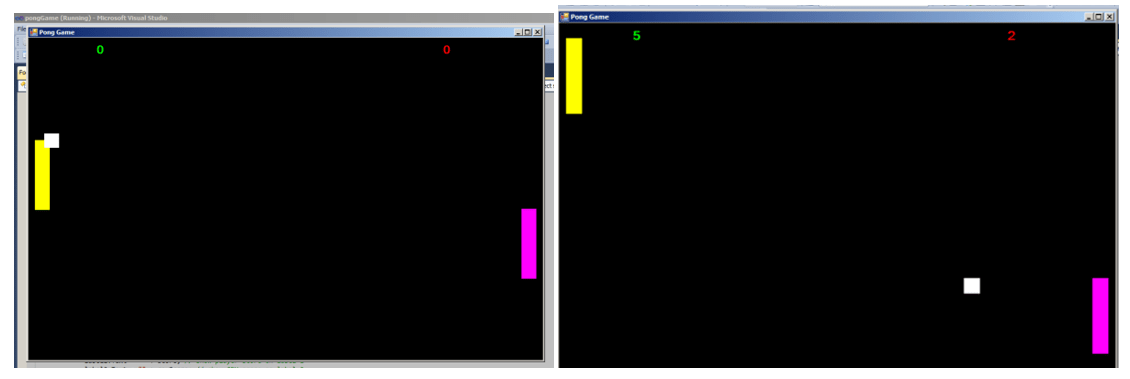Game is working fine. If you get any errors in the errors log, refer to this tutorial and see which line the error is coming up.

### 10 responses to “C# Tutorial – Create a Pong arcade game in Visual Studio”

1. ClashinMashing says:

the ball sometimes stick to the player

2. Foxtato says:

I can’t get the ball to move, eventhough my code is the same

3. EpsilonIT says:

What would you add to the code to make it where if someone resizes the game?

4. ozgr says:

How can ı use horizontal player?

5. Anhar Ali says:

@EpsilonIT you can change the form border style in the form properties window to fixed single or fixed dialog this will prevent anyone from resizing the window.

6. Anhar Ali says:

@ozgr if you want to make the game horizontal then you will need to use the player.Left and opponent.Left value instead of the Top value this will take the player and opponent side to side.

7. asdToi says:

IS IT POSIBLE IF YOU MAKE THE BALL CIRCLE?

8. Wasti says:

how do i make a AI that knows which directing the ball is moving so that it knows where to deflect.

9. Swampy says:

This was a lot of fun to make on my own. I’d been wanting to make a simple game to get some basic concepts. I appreciate you making this.

10. some C# beginner says:

Hi, It’s my first time here. I get the errors (CS0165: Use of unassigned local variable ‘goup/godown’). How do I fix it?